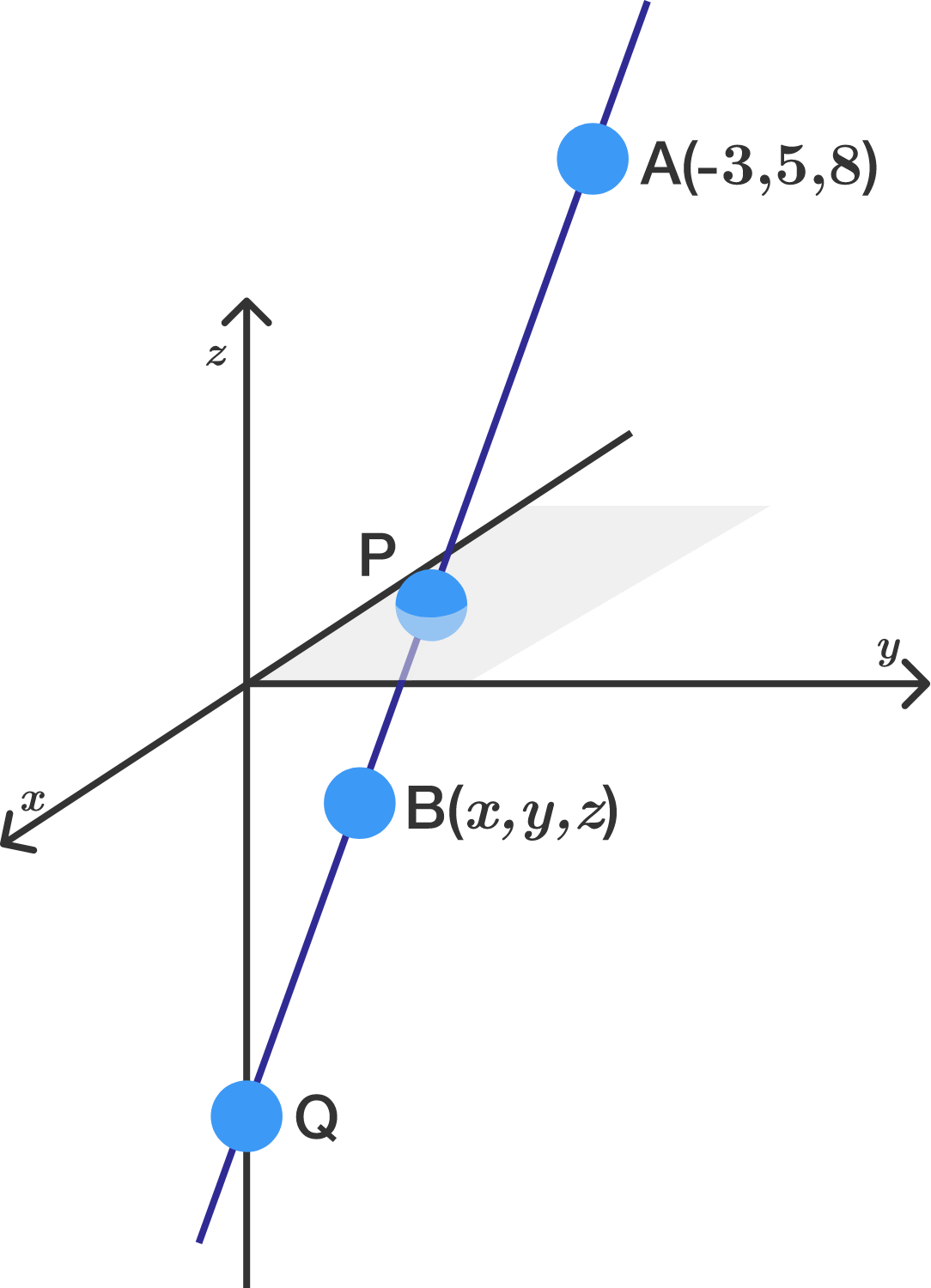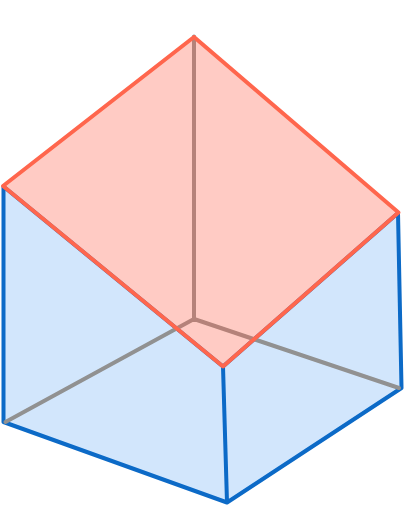Geometry

# 3D Coordinate Geometry: Level 2 Challenges

Point $P$ is some point on the surface of the sphere ${(x-1)}^{2}+{(y+2)}^{2}+{(z-3)}^{2}=1 .$ What is the shortest possible distance between $P$ and $O=(0, 0, 0)?$

If the two lines \begin{aligned} \frac {x-1 }{k } &=\frac { y+1 }{2 } =z,\\ \\ \\ \frac {x+2 }{-3 } & =1-y =\frac{z+2}{k} \end{aligned} are perpendicular to each other, then what is the value of $k?$

The points $A$, $B$, $P$, and $Q$ all lie on one line, with $A=(-3, 5, 8).$

Point $P$ lies in the $xy$-plane between $A$ and $B$ such that the distance from $A$ to $P$ is twice the distance from $P$ to $B$. Furthermore, $Q$ sits on the $z$-axis such that the distance from $A$ to $Q$ is twice the distance from $Q$ to $B$.

If $B=(x,y,z),$ what is the value of $x+y+z?$An infinite column is centered along the $z$-axis. It has a square cross-section of side length 10. It is cut by the plane $4x - 7y + 4z = 25.$

What is the area of the surface cut?

If the point $Q=(a, b, c)$ is the reflection of the point $P=(-6, 2, 3)$ about the plane $3x-4y+5z-9=0,$ determine the value of $a+b+c.$

×